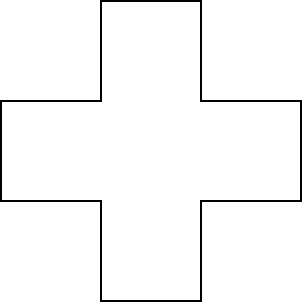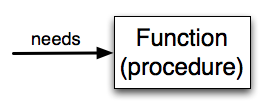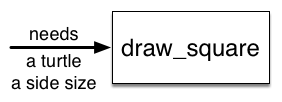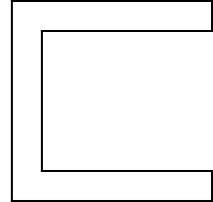# 3. Functions Introduction¶

Quick Overview of Day

Use for loops to draw shapes elegantly. Introduce non-fruitful functions in Python. Continue working on a Python turtle graphics assignment, focused on repetition and conditionals.

• CS20-CP1 Apply various problem-solving strategies to solve programming problems throughout Computer Science 20.
• CS20-FP1 Utilize different data types, including integer, floating point, Boolean and string, to solve programming problems.
• CS20-FP2 Investigate how control structures affect program flow.
• CS20-FP3 Construct and utilize functions to create reusable pieces of code.

## 3.1. Warmup Problem¶Draw the shape above. After you have finished drawing your version, share your code with another student. Work together to see if you can come up with a more efficient way to draw this shape!

## 3.2. Functions¶

In Python, a function is a named sequence of statements that belong together. Their primary purpose is to help us organize programs into chunks that match how we think about the solution to the problem.

The syntax for a function definition is the same as it was when we wrote functions in Reeborg:

def name( parameters ):
statements


The one part of this function definition that looks different from our Reeborg investigations is the parameters inside the parentheses. The parameters specify what information, if any, you have to provide in order to use the new function. Another way to say this is that the parameters specify what the function needs to do its work.

There can be any number of statements inside the function, but they have to be indented from the def. In the examples in this book, we will use the standard indentation of four spaces.

In a function definition, the keyword in the header is def, which is followed by the name of the function and some parameters enclosed in parentheses. The parameter list may be empty, or it may contain any number of parameters separated from one another by commas. In either case, the parentheses are required.

We need to say a bit more about the parameters. In the definition, the parameter list is more specifically known as the formal parameters. This list of names describes those things that the function will need to receive from the user of the function. When you use a function, you provide values to the formal parameters.

The figure below shows this relationship. A function needs certain information to do its work. These values, often called arguments or actual parameters, are passed to the function by the user.This type of diagram is often called a black-box diagram because it only states the requirements from the perspective of the user. The user must know the name of the function and what arguments need to be passed. The details of how the function works are hidden inside the “black-box”.

Suppose we’re working with turtles and a common operation we need is to draw squares. It would make sense if we did not have to duplicate all the steps each time we want to make a square. “Draw a square” can be thought of as an abstraction of a number of smaller steps. We will need to provide two pieces of information for the function to do its work: a turtle to do the drawing and a size for the side of the square. We could represent this using the following black-box diagram.Here is a program containing a function to capture this idea. Give it a try.

This function is named draw_square. It has two parameters — one to tell the function which turtle to move around and the other to tell it the size of the square we want drawn. In the function definition they are called some_turtle and side_length respectively. Make sure you know where the body of the function ends — it depends on the indentation and the blank lines don’t count for this purpose!

docstrings

If the first thing after the function header is a string (some tools insist that it must be a triple-quoted string), it is called a docstring and gets special treatment in Python and in some of the programming tools.

Another way to retrieve this information is to use the interactive interpreter, and enter the expression <function_name>.__doc__, which will retrieve the docstring for the function. So the string you write as documentation at the start of a function is retrievable by python tools at runtime. This is different from comments in your code, which are completely eliminated when the program is parsed.

By convention, Python programmers use docstrings for the key documentation of their functions.

Defining a new function does not make the function run. To do that we need a function call (also known as a function invocation). We’ve already seen how to call some built-in functions like print, range and int. Function calls contain the name of the function to be executed followed by a list of values in parentheses, called arguments, which are assigned to the parameters in the function definition. So in the last line of the program, we call the function, and pass alex as the turtle to be manipulated, and 50 as the size of the square we want.

Once we’ve defined a function, we can call it as often as we like and its statements will be executed each time we call it. In this case, we could use it to get one of our turtles to draw a square and then we can move the turtle and have it draw a different square in a different location. Note that we lift the tail so that when alex moves there is no trace. We put the tail back down before drawing the next square. Make sure you can identify both calls of the draw_square function.

In the next example, we’ve changed the draw_square function a little and we get tess to draw 15 squares with some variations. Once the function has been defined, we can call it as many times as we like with whatever actual parameters we like.

Warning

Even if a function call needs no arguments, the parentheses ( ) after the function name are required. This can lead to a difficult bug: A function name without the parenthesis is a legal expression referring to the function; for example, print and alex.penup, but they do not call the associated functions.

functions-intro1: Which of the following is a valid function header (first line of a function definition)?
• def draw_circle(my_turtle):
• A function may take zero or more parameters. It does not have to have two. In this case the size of the circle might be specified in the body of the function.
• def draw_circle:
• A function needs to specify its parameters in its header.
• draw_circle(my_turtle, side_length):
• A function definition needs to include the keyword def.
• def draw_circle(my_turtle, side_length)
• A function definition header must end in a colon (:).

functions-intro2: What are the parameters of the following function?

def draw_square(my_turtle, side_length):
"""Make turtle my_turtle draw a square of with side side_length."""
for i in range(4):
my_turtle.forward(side_length)
my_turtle.left(90)

• i
• i is a variable used inside of the function, but not a parameter, which is passed in to the function.
• my_turtle
• my_turtle is only one of the parameters to this function.
• my_turtle, side_length
• Yes, the function specifies two parameters: my_turtle and side_length.
• my_turtle, side_length, i
• the parameters include only those variables whose values that the function expects to receive as input. They are specified in the header of the function.

functions-intro3: Considering the function below, which of the following statements correctly calls, or invokes, this function (i.e., causes it to run)? Assume we already have defined a turtle named alex.

def draw_square(my_turtle, side_length):
"""Make turtle my_turtle draw a square of with side side_length."""
for i in range(4):
my_turtle.forward(side_length)
my_turtle.left(90)

• def draw_square(my_turtle, side_length)
• No, my_turtle and side_length are the names of the formal parameters to this function. When the function is called, it requires actual values to be passed in.
• draw_square
• A function call always requires parentheses after the name of the function.
• draw_square(10)
• This function takes two parameters (arguments)
• draw_square(alex, 10):
• A colon is only required in a function definition. It will cause an error with a function call.
• draw_square(alex, 10)
• Since alex was already previously defined and 10 is a value, we have passed in two correct values for this function.
functions-intro4: True or false: A function can be called several times by placing a function call in the body of a loop.
• True
• Yes, you can call a function multiple times by putting the call in a loop.
• False
• One of the purposes of a function is to allow you to call it more than once. Placing it in a loop allows it to executed multiple times as the body of the loop runs multiple times.

## 3.3. Practice Problems¶

### 3.3.1. Cross¶Convert the code you wrote at the start of today’s class into a function. You should be able to call something like draw_cross(some_turtle, side_length).

### 3.3.2. Hollow C¶Look back to the code you made when you drew a hollow c last class. Adapt your code by creating a function called draw_c(a_turtle, longest_side_length, width_of_c). You should be able to draw a hollow c by calling the function with something like draw_c(tess, 150, 25).

## 3.4. Turtle Graphics Assignment¶

Use the rest of this class time to keep working on your current Python assignment (possibly a turtle graphics drawing, with a focus on looping and conditionals).

Next Section - 4. Turtle Drawing Practice